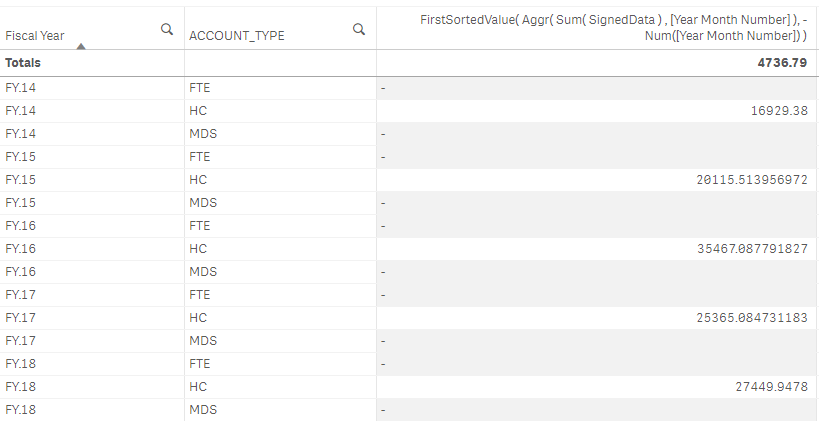# Qlik Design Blog

All about product and Qlik solutions: scripting, data modeling, visual design, extensions, best practices, etc.

Announcements
QlikWorld Online 2021, May 10-12: Our Free, Virtual, Global Event REGISTER TODAYEmployee

## Additive and Non-Additive Numbers

Facts in BI solutions are usually additive – but not always. To avoid mistakes, it is important to understand when you can sum the number, and when you cannot. This post will not only help you understand the problem, but also point at some possible ways to handle non-additive numbers.

Numbers fall into one of three categories:

• Fully additive numbers, which can be summed across any of the dimensions. Most transactional amounts are additive.
• Semi-additive numbers, which can be summed across some dimensions, but not all. An example is warehouse balances: these are amounts that are additive across all dimensions except time.
• Non-additive numbers, which cannot be summed over any dimension. An example is the gross margin for a product.

Additive numbers are straightforward: Just use the Sum() function in your measure, and everything will work.

Semi-additive and non-additive numbers are however not as straightforward. These will be shown correctly only in a chart with exactly the same grain as the source data, i.e. if the chart dimension(s) is the primary key in the source table. In all other cases the sum function will potentially return incorrect results. In other words - these numbers are often unusable in a dynamic analysis app.

But there are ways to get around the problem:

Balances

Balances and other numbers describing a situation in time are usually semi-additive. They can be summed over all dimensions, except time. Adding the balance for one month with that of the next month would not make sense. But it does make sense to sum balances from different warehouses. So for balances you need to adapt the expression to reflect this. If your data contains one balance number per month, you can often use an expression similar to one of the following:

Average:      Sum( Balance ) / Count( distinct Month )

Last value:   FirstSortedValue( Aggr( Sum( Balance ) , Month ), -Month )

In the table below, you can see the population for some countries during four years. By dividing by the number of years, the semi-additive population can be used also in the total column.Ratios

Ratios, percentages and averages are usually non-additive numbers. Examples are the gross margin and the average order value. If you have such fields in your source data, it is likely that your data already has been aggregated once. Such fields are often calculated by dividing one number with another, e.g. by expressions similar to the following:

GrossMargin           = ( Sum ( Revenue ) - Sum( CostOfGoods ) ) / Sum( Revenue )

AverageOrderValue  = Sum ( OrderValue )  / Count ( distinct OrderID )

Note that the numerators and the denominators all are additive numbers!

Hence, if you have the original numerator and the denominator in your source data, you should use these in your application, instead of the pre-calculated ratios. In other words: Use the above expressions as measures in charts or gauges to define your gross margin and average order value. Do not use the pre-calculated ratios.

Mixed Units

In some cases, you have mixed units in a field, which makes the numbers non-additive. The most common case is that you have mixed currencies. This is a problem that is easily solved: Just convert the numbers to a common currency or common unit already in the script, by multiplying with the appropriate currency rate, e.g.

LocalCurrency * CurrencyRate as CommonCurrency,

Then you can use the common currency as an additive field. You may need a join or an Applymap() to get the correct currency rate into the fact table.

Incomplete Data

There is also the case of incomplete data, i.e. where you have the total number and the numbers for some parts, but data is missing for some other parts. In such a case, you need to convert this source table to a complete set of data, e.g. by removing the "Total" record and introducing an "Others" record that is calculated from the total minus the sum of all known parts.

Bottom line: If you have semi-additive or non-additive numbers, you need to convert these to something usable using one of the methods described here. If you can't, the numbers are almost useless and you should use the Only() function when you display them, thereby preventing a summation.

HIC

Further reading related to this topic:

Scales of Measurement

7 CommentsMVP & Luminary

Another good article HIC.  Thanks for sharing.

Regards,

Jagan.

1,918 ViewsNot applicable

Really good article and interesting topic

-Mika

1,918 ViewsNot applicable

Good Post Henric ...

1,918 ViewsLuminary

I also call these non-additive cases non-summation aggregations rules1,918 ViewsContributor III

hello everyone!

I have a question about handling semi additive measures in qlikview,I didn't figure out how to handle these measures when there is more than one balance per month (for each product,store,..)

kindly please help me out of this. Thanks

Additive and Non-Additive Numbers

1,918 ViewsPartner

I am trying this solution on my end and it worked when i am breaking them down with the fields on the Calendar dimension.

But when i add field from other Dims only the value that is also FirstSortOrder gets a value while rest are Null:

Measure: FirstSortedValue( Aggr( Sum( SignedData ) , [Year Month Number] ), -Num([Year Month Number] ) )848 Views